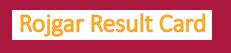Simple Formulae For CGPA to Percentage Calculator Conversion Online - Rojgarresultcard.com | Rojgar Result | Lotto Result | Online Form 2021Rojgar Result Card: RojgarResultCard.Com, Provide Official Rojgar Results 2021, Sarkari Lotto Result 2021, Latest Government Jobs, Admit Card, Answer Key, admission news, merit list, counseling and answer key.

What is Easy Formulae To Convert CGPA to Percentage Calculator Online?
Answer: The Formula CGPA to % Conversion is to get percentage to CGPA convertor software Download CGPA % Calculator Formulae as this portal is dedicated to give how to convert CGPA conversion to Percentage calculations online. As now it is very easy to convert CGPA to % Formula Calculator from this page.  In our previous article we have already explain CGPA full form and now teachers will be able to understand the process of calculate percentage from cgpa.  The grade point and percentage of marks will be accessed in 02 different ways through grading pattern and cgpa.  Further more information of Convert CGPA to Percentage Calculator regarding CBSE Grade System Formula, shortcut tricks and conversion process etc is mentioned below………………………

### Details of CGPA to Percentage Calculator:

This method is used by CBSE to calculate overall indicative percentage by multiply CGPA with 9.5.  In this board took the results of the last 05 years and calculate the average marks of all candidates who had scored between 91 and 100 and that average is approx 95 marks.  The equivalent Grade Point for the 91 - 100 marks range is A1 grade of marks is 10, it then divided the average result of 95 by 10. The result is 9.5.
 Formulae CGPA to % Calculator Domain - Category GK Tricks Mode Online Status Available

### >> How to Convert CGPA to Percentage Calculator?

Answer: There are 02 methods to get percentage from cgpa issued by CBSE.  These are as follows: -
a) Subject wise indicative percentage of marks = 9.5 x GP (Grade Point) of the Subject
b) Overall indicative percentage of marks = 9.5 x CGPA

>> CGPA Formula Percentage Calculate Format:
 Class 9 c Subject: a/b/c Term I Sno. Name of student FA1 (10) G FA2 (10) G SA1 (30) G T1 (50) % G 1 Vikram 9.5 A1 9 A2 26 A2 44.5 89 A2 Class 9 c Subject: a/b/c Term II Sno. Name of student FA1 (10) G FA2 (10) G SA2 (30) G T2 (50) % G 1 Vikram 7 B2 7 B2 24 B1 38 76 B1 Class 9 c Subject: a/b/c Total = T1 + T2 Sno. Name of student (50) T1 (50) T2 100 G.T F.G 1 Vikram 44.5 38 82.5 A2
It will be much easier for you by view image given below…………………………….

The above formula given by CBSE to convert grades into marks and percentage is based on approximation and not exact figures...

Thanks & Regards,